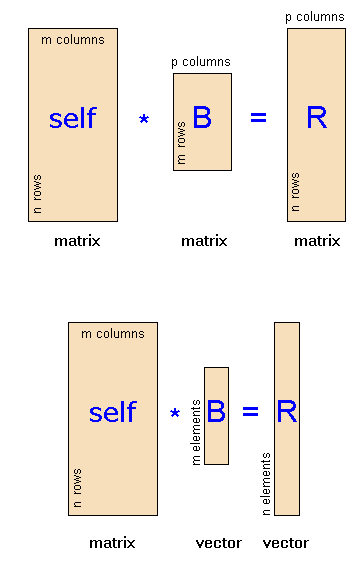The SDL Component Suite is an industry leading collection of components supporting scientific and engineering computing. Please visit the SDL Web site for more information....## Multiply

 Unit: SDL_matrix Class: Declaration:  function Multiply (MatB, MatRes: TMatrix): boolean; { class TMatrix }  function Multiply (VecB, VecRes: TVector): boolean; { class TMatrix }  function Multiply (MatB, MatRes: TIntMatrix): boolean; { class TIntMatrix }  function Multiply (VecB, VecRes: TIntVector): boolean; { class TIntMatrix }

The function Multiply calculates the product of matrix self with either the matrix MatB (version ) or the vector VecB (version ). The results are stored in MatRes or VecRes, respectively. The function returns the value TRUE, if the multiplication has been carried out successfully. The second overloaded method multiplies the matrix with the vector VecB resulting in the vector VecRes.

 Hint: The multiplication of two matrices is only possible if the number of columns of the first matrix (self ) equals the number of rows of the second matrix (MatB ). The resulting matrix MatRes or the resulting vector VecRes are automatically resized to the appropriate dimensions. The following figure illustrates the context:Example: The statement MatA.Multiply (MatB, MatR) multiplies the matrix MatA with MatB and stores the result in MatR.

Last Update: 2016-Jun-28